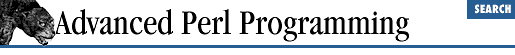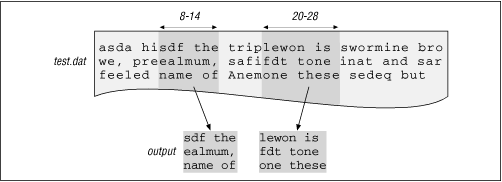Chapter 5Eval5.5 Using Eval for Efficiency

Here are some examples where run-time evaluation can greatly speed up execution.

5.5.1 A Fast Multipattern grep

Consider a grep-like Perl script that can search for any number of patterns and print out only those lines that match all the given patterns (the order of the patterns being unimportant). You might structure the code like this:

while (\$s = <>) {
\$all_matched = 1;     # start by assuming all patterns match \$s
foreach \$pat (@patterns) {
if (\$s !~ /\$pat/) {
\$all_matched = 0; # No, our assumption was wrong
last;
}
}
print \$s if \$all_matched;
}

The problem with this code is that the regular expression (/\$pat/) is compiled afresh for every line and for every pattern. That is, if you have, say, 10,000 lines in the text to be searched, and three patterns to search for, a.*b, [0-9], and [^def], the patterns will be compiled 30,000 times. The /o flag, which tells Perl to compile the regular expression, cannot be used here because \$pat can vary as the program executes.

The fastest approach would be to hardcode the patterns as shown next. Unfortunately, it is also the least reusable approach.

while (\$s = <> ) {
if (  (\$s =~ /a.*b/) &&
(\$s =~ /[0-9]\$/) &&
(\$s =~ /[^def]/)) {
print \$s;
}
}

The good news is that it is possible to get this level of efficiency without losing generality. The idea is to hand-craft the "hard-wired" code above at run-time and then to eval it.

The strings constituting the code to be generated are shown in bold in Example 5.2.

Example 5.2: Compiling Regular Expression Strings for Blazing Speed

\$code = 'while (<>) {';
\$code .= 'if (/';
\$code .= join ('/ && /', @patterns);
\$code .= '/) {print \$_;}}';
print \$code, "\n";
eval \$code;   # Ahh, finally !
# Check if faulty regular expressions given as input patterns
die "Error ---: \$@\n Code:\n\$code\n"    if (\$@);

If @patterns contains the three strings "^abc", "ghi", "efg\$", for example, the code supplied to eval looks like this:

while (<>) {if (/^foo/ && /bar\$/ && /ghi/) {print \$_;}}

One way to make this example even more efficient is to sort the patterns such that those patterns with beginning- or end-of-line markers (^ and \$) are evaluated first. It is much faster to check for a pattern anchored to the beginning or end of the line than to search all over the string. Another enhancement to make is for the user to supply the Boolean operator instead of hardcoding &&. See the perlfaq6 document (FAQ on regular expressions) that is bundled with the Perl distribution.

5.5.2 Extracting Columns from a File

Let us look at another example that builds and evaluates Perl code dynamically, for efficiency. We build a program called col, which extracts columns from a file, similar to the Unix cut(1) command. It is invoked as shown below:

% col -s80 8-14 20+8 test.dat

This invocation treats test.dat as a fixed-format file with 80-column records and extracts two columns from each record, one starting at character position 8 and ending at 14 (the index of the leftmost column is 1, not 0) and the other going from 20 through 28, as shown in Figure 5.2. If the -s option is not given, the script treats newlines as record terminators and reads the file line by line. col allows column ranges to overlap.

Figure 5.2: Using col for extracting columnsYou already know that substr extracts substrings given a starting location and the substring length. Writing col is a simple matter of calling substr in a loop, once for each range given on the command line:

for each line in the file {
for each column range in the command line arguments {
print substr (line, range);
}
}

As an aside, we don't use unpack instead of substr because we would like the input ranges to overlap.

A more efficient alternative to the preceding solution is to "flatten the loop" and use constants wherever possible, as shown in the following code snippet (for the specific command-line invocation above). For each record read from a file, this code extracts the substring indicated by input range and pads it with spaces as necessary. It also appends a delimiter ("|") to each extracted column.

#PART 1 ---------------------------------------------------------
sub col {
my \$tmp;
while (1) {
\$s = get_next_line();
\$col = "";

#PART 2 ----------------------------------------------------------
\$s .= ' ' x (14 - length(\$s))  if (length(\$s) < 14);
\$tmp = substr(\$s, 7, 7);
\$tmp .= " " x (7 - length(\$tmp));
\$col .= '|' . \$tmp;
\$s .= ' ' x (28 - length(\$s))    if (length(\$s) < (28));
\$tmp = substr(\$s, 19, 9);
\$tmp .= " " x (9 - length(\$tmp));
\$col .= '|' . \$tmp;

#PART 3 ----------------------------------------------------------
print \$col, "\n";
}
}

\$tmp contains one column at any time, and \$col accumulates each such column to be output and is finally printed.

Given the command line as shown, let's compose this subroutine at run-time. Notice that parts 1 and 3 are independent of the command-line arguments. Part 2, which extracts all the columns in each line, is the only one that is shaped by command-line arguments.

As was mentioned earlier, you must watch your quotes carefully. Assume that \$col1 and \$offset hold 7 and 6, respectively, so that we need to insert this line of code into our executable string:

\$tmp = substr(\$s, 7, 6);

Here is how we can generate this line:

\$code = '\$tmp = substr(\$s, ' . "\$col1, \$offset)";

Note how we use single and double quotes to carefully control variable interpolation. Example 5.3 shows the three parts being generated by generate_part1, generate_part2, and generate_part3. The subroutine get_next_line converts tabs to equivalent spaces to preserve the visual effect of a tab. generate_part3 also evals this generated code and introduces the new subroutine col. As before, the strings representing the code are shown in bold lettering.

Example 5.3: col: A Script to Extract Columns from a File

# Extracts columns of text from a file
# Usage : col [-s<n>] col-range1, col-range2, files ...
# where col-range is specified as col1-col2 (column 1 through column2)
#         or col1+n, where n is the number of columns.
\$size = 0;          # 0 => line-oriented input, else fixed format.
@files = ();        # List of files
\$open_new_file = 1; # force get_next_line() to open the first file
\$debugging = 0;     # Enable with -d commmand line flag
\$col = "";
\$code = "";
generate_part1();
generate_part2();
generate_part3();
col();           # sub col has now been generated. Call it !
exit(0);

#------------------------------------------------------------------
sub generate_part1 {
# Generate the initial invariant code of sub col()
\$code  = 'sub col { my \$tmp;';           # Note the single quotes
\$code .= 'while (1) {\$s = get_next_line(); \$col = "";';
\$delimiter = '|';
}

#------------------------------------------------------------------
sub generate_part2 {
# Process arguments
my (\$col1, \$col2);
foreach \$arg (@ARGV) {
if ((\$col1, \$col2) = (\$arg =~ /^(\d+)-(\d+)/)) {
\$col1--;# Make it 0 based
\$offset = \$col2 - \$col1;
} elsif ((\$col1, \$offset) = (\$arg =~ /^(\d+)\+(\d+)/)) {
\$col1--;
} elsif (\$size = (\$arg =~ /-s(\d+)/)) {
# noop
} elsif (\$arg =~ /^-d/) {
\$debugging = 1;
} else {
# Must be a file name
push (@files, \$arg);
}
}
}

#------------------------------------------------------------------
sub generate_part3 {
\$code .= 'print \$col, "\n";}}';

print \$code if \$debugging; # -d flag enables debugging.
eval \$code;
if (\$@) {
die "Error ...........\n \$@\n \$code \n";
}
}

#------------------------------------------------------------------
my (\$col1, \$numChars) = @_;
# substr complains (under -w) if we look past the end of a string
# To prevent this, pad the string with spaces if necessary.
\$code .= "\\$s .= ' ' x (\$col1 + \$numChars - length(\\$s))";
\$code .= "    if (length(\\$s) < (\$col1+\$numChars)
);";
\$code .= "\\$tmp = substr(\\$s, \$col1, \$numChars);";
\$code .= '\$tmp .= " " x (' . \$numChars .  ' - length(\$tmp));';
\$code .= "\\$col .= '\$delimiter' . \\$tmp; ";
}

#------------------------------------------------------------------
sub get_next_line {
my(\$buf);

NEXTFILE:
if (\$open_new_file) {
\$file = shift @files || exit(0);
open (F, \$file) || die "\$@ \n";
\$open_new_file = 0;
}
if (\$size) {
} else {
\$buf = <F>;
}
if (! \$buf) {
close(F);
\$open_new_file = 1;
goto NEXTFILE;
}
chomp(\$buf);
# Convert tabs to spaces (assume tab stop width == 8)

# expand leading tabs first--the common case
\$buf =~ s/^(\t+)/' ' x (8 * length(\$1))/e;

# Now look for nested tabs. Have to expand them one at a time - hence
# the while loop. In each iteration, a tab is replaced by the number of
# spaces left till the next tab-stop. The loop exits when there are
# no more tabs left
1 while (\$buf =~ s/\t/' ' x (8 - length(\$`)%8)/e);

\$buf;
}

get_next_line uses the substitute operator's /e option to remove tabs. Can you guess why we have to use the while loop instead of the /g option? The reason is that to expand a tab to the correct number of spaces, we have to know where the tab and the next tab stop are located. This means we have to know the number of characters from the beginning of the line to the tab, which is obtained by computing length(\$`). In the next iteration, this length needs to account for the previously expanded tab. While /g does a global replace, it never revisits a substituted portion (that is, it always moves forward), with the result that by using this option, you can never find out how long the partially substituted string is at any point. Instead, we use the while loop to traverse the string from the beginning for each tab found.5.4 Using Eval for Expression Evaluation5.6 Using Eval for Time-Outs